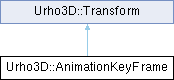Rebel Fork Framework
Urho3D::AnimationKeyFrame Struct Reference

`#include <Urho3D/Graphics/AnimationTrack.h>`

Inheritance diagram for Urho3D::AnimationKeyFrame:## Public Member Functions

AnimationKeyFrame (float time, const Vector3 &position, const Quaternion &rotation=Quaternion::IDENTITY, const Vector3 &scale=Vector3::ONE)Public Member Functions inherited from Urho3D::Transform
Matrix3x4 ToMatrix3x4 () const
Construct Matrix3x4 from Transform.

Transform Lerp (const Transform &rhs, float t) const
Interpolate between two transforms.

Transform Inverse () const
Return inverse transform. It is not precise for non-uniform scale.

Transform operator* (const Transform &rhs) const
Return transform multiplied by another transform.

Vector3 operator* (const Vector3 &rhs) const
Return position multiplied by the transform.

Quaternion operator* (const Quaternion &rhs) const
Return rotation multiplied by the transform.

## Public Attributes

float time_ {}
Keyframe time.Public Attributes inherited from Urho3D::Transform
Vector3 position_

Quaternion rotation_

Vector3 scale_ {Vector3::ONE}Static Public Member Functions inherited from Urho3D::Transform
static Transform FromMatrix3x4 (const Matrix3x4 &matrix)
Construct Transform from Matrix3x4. It is not precise for non-uniform scale.Static Public Attributes inherited from Urho3D::Transform
static const Transform Identity {Vector3{}, Quaternion{}, Vector3{1.0f, 1.0f, 1.0f}}

## Detailed Description

Skeletal animation keyframe. TODO: Replace inheritance with composition?

The documentation for this struct was generated from the following file: